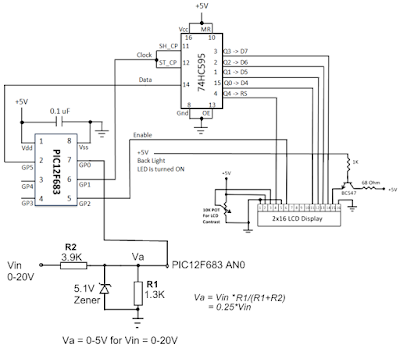### pic12f683 ile voltmetre 0-20 volt0-20 volt ölçüm için tasarlanmış devrede, pic12f683 ölçüm giriş voltajı 0-5 volt. bilindiği gibi pic ile en fazla 5 volt ölçüm yapabiliyoruz. Aşağıdaki basit ölçüm devresi ile 20 volt giriş 5 volt seviyesine indirilerek ölçüm sağlanmış. Kaynak siteye buradan gidebilirsiniz.

Ölçüm devresi:Devre şeması :C kaynak kodu:

/* Project: 0-20V Digital Voltmeter using PIC12F683
Uses the 3-wire Serial LCD display from previous project to display voltage
Rajendra Bhatt, Oct 3, 2010
*/

sbit Data_Pin at GP5_bit;
sbit Clk_Pin at GP1_bit;
sbit Enable_Pin at GP2_bit;

// Always mention this definition statement
unsigned short Low_Nibble, High_Nibble, p, q, Mask, N,t, RS, Flag, temp;

void Delay_50ms(){
Delay_ms(50);
}

void Write_LCD_Nibble(unsigned short N){
Enable_Pin = 0;
// ****** Write RS *********
Clk_Pin = 0;
Data_Pin = RS;
Clk_Pin = 1;
Clk_Pin = 0;
// ****** End RS Write

// Shift in 4 bits
}
// One more clock because SC and ST clks are tied
Clk_Pin = 1;
Clk_Pin = 0;
Data_Pin = 0;
Enable_Pin = 1;
Enable_Pin = 0;
}
// ******* Write Nibble Ends

void Write_LCD_Data(unsigned short D){
RS = 1; // It is Data, not command
Low_Nibble = D & 15;
High_Nibble = D/16;
Write_LCD_Nibble(High_Nibble);
Write_LCD_Nibble(Low_Nibble);
}

void Write_LCD_Cmd(unsigned short C){
RS = 0; // It is command, not data
Low_Nibble = C & 15;
High_Nibble = C/16;
Write_LCD_Nibble(High_Nibble);
Write_LCD_Nibble(Low_Nibble);
}

void Initialize_LCD(){
Delay_50ms();
Write_LCD_Cmd(0x20); // Wake-Up Sequence
Delay_50ms();
Write_LCD_Cmd(0x20);
Delay_50ms();
Write_LCD_Cmd(0x20);
Delay_50ms();
Write_LCD_Cmd(0x28); // 4-bits, 2 lines, 5x7 font
Delay_50ms();
Write_LCD_Cmd(0x0C); // Display ON, No cursors
Delay_50ms();
Write_LCD_Cmd(0x06); // Entry mode- Auto-increment, No Display shifting
Delay_50ms();
Write_LCD_Cmd(0x01);
Delay_50ms();
}

void Position_LCD(unsigned short x, unsigned short y){
temp = 127 + y;
if (x == 2) temp = temp + 64;
Write_LCD_Cmd(temp);
}

void Write_LCD_Text(char *StrData){
q = strlen(StrData);
for (p = 0; p
temp = StrData[p];
Write_LCD_Data(temp);
}

}

char Message1[] = "DVM Project";
char *volt = "00.0";

void main() {
CMCON0 = 7; // Disable Comparators
TRISIO = 0b00001001; // All Outputs, except GP0 and GP3
ANSEL = 0x01; // GP0 analog i/p

Initialize_LCD();
Position_LCD(1,3);
Write_LCD_Text(Message1);
Position_LCD(2,10);
Write_LCD_Data('V');

do {

volt = DisplayVolt/1000 + 48;
volt = (DisplayVolt/100)%10 + 48;
volt = (DisplayVolt/10)%10 + 48;
Position_LCD(2,5);
Write_LCD_Text(volt);
delay_ms(100);
} while(1);

}

### Yorumlar

1.Pic16f683 değil hocam pic12f683 olacak..

2.Sağolun uyarınız için hemen düzeltiyorum.